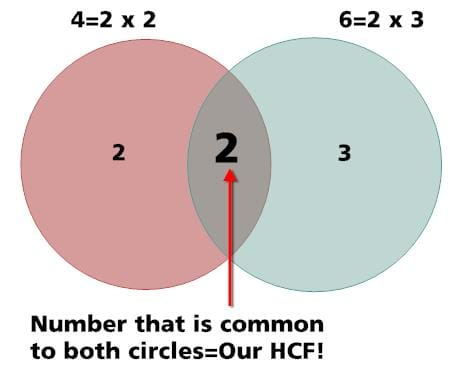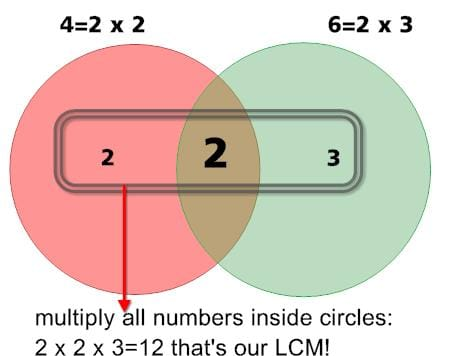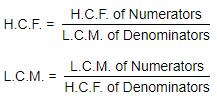CAT  >  Introduction & Concept: HCF & LCM

# Introduction & Concept: HCF & LCM - Notes | Study Quantitative Aptitude (Quant) - CAT

 Table of contentsIntroductionWhat is Highest Common Factor (H.C.F.)?Finding the GCD / H.C.F. of two numbers E and RWhat is Least Common Multiple (L.C.M.)?HCF and LCM FormulaH.C.F. and L.C.M. of Decimal FractionsComparison of FractionsSolved Questions1 Crore+ students have signed up on EduRev. Have you?

Introduction

• The chapter of HCF & LCM is part of the Number Systems unit which is the most important chapter in the entire syllabus of Quantitative Aptitude for the CAT examination (and also for other parallel MBA entrance exams).
• Students are advised to go through this chapter with utmost care understanding each concept and question type on this topic. The CAT has consistently contained anything between 20-40% of the marks based on questions taken from this chapter.

What is Highest Common Factor (H.C.F.)?

• H.C.F is also called as Greatest Common Measure (G.C.M.) or Greatest Common Divisor (G.C.D).
• The H.C.F. of two or more than two numbers is the greatest number that divides each of them exactly.

Finding the GCD / H.C.F. of two numbers E and R

• Find the standard form of the numbers E and R.
• Write out all prime factors that are common to the standard forms of the numbers E and R.
• Raise each of the common prime factors listed above to the lesser of the powers in which it appears in the standard forms of the numbers E and R.
• The product of the results of the previous step will be the GCD of E and R.
• H.C.F of (4,6):Example 1: Find the GCD of 150, 210, 375.

• Step 1: Writing down the standard form of numbers
⇨ 150 = 5 X 5 X 3 X 2
⇨ 210 = 5 X 2 X 7 X 3
⇨ 375 = 5 X 5 X 5 X 3
• Step 2: Writing Prime factors common to all the three numbers is 5 X 3.
• Step 3: Hence, the HCF will be 5 X 3 = 15.

Methods of finding the HCF

(i) Factorization Method (Prime Factorisation Method)

• Step 1: Express each one of the given numbers as the product of prime factors.
• Step 2:The product of the least powers of common prime factors gives H.C.F.

(ii) Division Method

• Step 1: Divide the larger number by the smaller number and obtain the remainder.
• Step 2: Divide the divisor by the remainder obtained in Step 1.
• Step 3: Repeat the process of dividing the preceding divisor by the remainder last obtained till you get 0 as remainder.
• Step 4: The last divisor is the required H.C.F.

Shortcut Method for Finding the HCF

• The above-mentioned illustration is however extremely cumbersome and time taking. Let us look at a much faster way of finding the HCF of a set of numbers.

Suppose you were required to find the HCF of 39,78 and 195:

• Logic:  The HCF of these numbers would necessarily have to be a factor (divisor) of the difference between any pair of numbers from the above 3.
The HCF has to be a factor of (78 – 39 = 39) as well as of (195 – 39 = 156) and (195 – 78 = 117). Why? Well, the logic is simple if you were to consider the tables of numbers on the number line.
• For any two numbers on the number line, a common divisor would be one which divides both. However, for any number to be able to divide both the numbers, it can only do so if it is a factor of the difference between the two numbers. Got it?? No??
• Then, Let’s see an illustrative:
Say we take the numbers 68 and 119. The difference between them being 51, it is not possible for any number outside the factor list of 51 to divide both 68 and 119. Thus, for example, a number like 4, which divides 68 can never divide any number which is 51 away from 68 because 4 is not a factor of 51. Only factors of 51, i.e. 51,17,3 and 1 ‘could’ divide both these numbers simultaneously.

Example 2: The sides of a hexagonal field are 216, 423, 1215, 1422, 2169 and 2223 metres. Find the greatest length of tape that would be able to exactly measure each of these sides without having to use fractions/parts of the tape?

Before solving this example, remind yourself with the above short trick you read above.
⇨ In this question, we are required to identify the HCF of the numbers 216, 423,1215, 1422, 2169 and 2223.
⇨ In order to do that, we first find the smallest difference between any two of these numbers. It can be seen that the difference between 2223-2169 = 54.

Thus, the required HCF would be a factor of the number 54. The factors of 54 are: 1 × 54, 2 × 27, 3 X 18, 6 X 9

⇨ One of these 8 numbers has to be the HCF of the 6 numbers. 54 cannot be the HCF because the numbers 423 and 2223 being odd numbers would not be divisible by any even number. Thus, we do not need to check any even numbers in the list.

⇨ 27 does not divide 423 and hence cannot be the HCF. 18 can be skipped as it is even.

Checking for 9: 9 divides 216,423,1215,1422 and 2169.

⇨ Hence, it would become the HCF. (Note: we do not need to check 2223 once we know that 2169 is divisible by 9)

Question for Introduction & Concept: HCF & LCM
Try yourself:A nursery has 363,429 and 693 plants respectively of 3 distinct varieties. It is desired to place these plants in straight rows of plants of 1 variety only so that the number of rows required is the minimum. What is the size of each row and how many rows would be required? (Try to solve using the shortcut method)?

What is Least Common Multiple (L.C.M.)?

LCM stands for Lowest or Least Common Multiple. The LCM of two or more numbers is the smallest positive integer that is divisible by all the given numbers.

Finding the LCM two numbers E and R

• Find the standard form of the numbers E and R.
• Write out all the prime factors, which are contained in the standard forms of either of the numbers.
• Raise each of the prime factors listed above to the highest of the powers in which it appears in the standard forms of the numbers E and R.
• The product of the results of the previous step will be the LCM of E and R.
• L.C.M of (4,6) is:Example 3: Find the LCM of 150, 210, 375.

• Step 1: Writing down the standard form of numbers:
⇨ 150 = 5 × 5 × 3 × 2 = 5× 3 × 2
⇨ 210 = 5 × 2 × 7 × 3
⇨ 375 = 5 × 5 × 5 × 3 = 53 × 3
• Step 2: Write down all the prime factors: that appear at least once in any of the numbers: 5, 3, 2, 7.
• Step 3: Raise each of the prime factors to their highest available power (considering each to the numbers).
The LCM = 2 × 3 × 5 × 5 × 5 × 7 = 5250.

Methods of finding the LCM

(i) Factorization Method

• Step 1: Express each of the given numbers as the product of prime factors.
• Step 2: The product of terms containing the highest powers of all the factors gives the L.C.M. of the given numbers.

(ii) Division Method (short-cut)

• Step 1: Arrange the given number in a row.
• Step 2: Divide by a number that divides exactly at least two of the given numbers and carry forward the numbers which are not divisible.
• Step 3: Repeat the above process till no two numbers are divisible by the same number except 1.
• Step 4: The product of the divisors and the undivided numbers is the required L.C.M. of the given numbers.

Question for Introduction & Concept: HCF & LCM
Try yourself:The LCM of two numbers is 936. If their HCF is 4 and one of the numbers is 72, the other is:

Example 4: Find the L.C.M. of 72, 240, 196.

(i) Using Prime factorisation method:

⇨ 72 = 2 × 2 × 2 × 3 × 3 = 2× 32
⇨ 240 = 2 × 2 × 2 × 2 × 3 × 5 = 2× 3 × 5
⇨ 196 = 2 × 2 × 7 × 7 = 2× 72

L.C.M. of the given numbers = Product of all the prime factors of each of the given number with greatest index of common prime factors
= 2× 3× 5 × 72 = 16 × 9 × 5 × 49 = 35280.

(ii) Using the Division method:
2 | 72, 240, 196
2 | 36, 120, 98
2 | 18, 60 , 49
3 | 9 , 30 , 49
L.C.M. of the given numbers:
= Product of divisors and the remaining numbers
= 2 × 2 × 2 × 3 × 3 × 10 × 49
= 35280

Question for Introduction & Concept: HCF & LCM
Try yourself:Find Greatest Number, which will divide 215,167 and 135 so as to leave the same remainder in each case

Co-primes: Two numbers are said to be co-primes if their H.C.F. is 1.HCF and LCM Formula

The formula which involves both HCF and LCM is:

Product of Two numbers = (HCF of the two numbers) x (LCM of the two numbers)

GCD (E, R) × LCM (E, R) = E × R

Note: This rule is applicable only for two numbers

H.C.F. and L.C.M. of Decimal Fractions

In given numbers, make the same number of decimal places by annexing zeros in some numbers, if necessary. Considering these numbers without a decimal point, find H.C.F. or L.C.M. as the case may be. Now, in the result, mark off as many decimal places as are there in each of the given numbers.

Comparison of Fractions

Find the L.C.M. of the denominators of the given fractions. Convert each of the fractions into an equivalent fraction with L.C.M as the denominator, by multiplying both the numerator and denominator by the same number. The resultant fraction with the greatest numerator is the greatest.

Question for Introduction & Concept: HCF & LCM
Try yourself:What Will Be The Least Possible Number Of The Planks, if three pieces of timber 42 m, 49 m, and 63 m long have to be divided into planks of the same length?

Solved Questions

Q.1. How many pairs of integers (x, y) exist such that the product of x, y and HCF (x, y) = 1080?

1. 8
2. 7
3. 9
4. 12

Solution:

We need to find ordered pairs (x, y) such that xy * HCF(x, y) = 1080.
Let x = ha and y = hb where h = HCF(x, y) => HCF(a, b) = 1.
So h3(ab) = 1080 = (23)(33)(5).
We need to write 1080 as a product of a perfect cube and another number.
Four cases:
1. h = 1, ab = 1080 and b are co-prime. We gave 4 pairs of 8 ordered pairs (1, 1080), (8, 135), (27, 40) and (5, 216). (Essentially we are finding co-prime a,b such that a*b = 1080).
2. h = 2, We need to find a number of ways of writing (33) * (5) as a product of two co-prime numbers. This can be done in two ways - 1 and (33) * (5) , (33) and (5)
number of pairs = 2, number of ordered pairs = 4
3. h = 3, number of pairs = 2, number of ordered pairs = 4
4. h = 6, number of pairs = 1, number of ordered pairs = 2
Hence total pairs of (x, y) = 9, total number of ordered pairs = 18.
The pairs are (1, 1080), (8, 135), (27, 40), (5, 216), (2, 270), (10, 54), (3, 120), (24, 15) and (6, 30).

Q.2. Find the smallest number that leaves a remainder of 4 on division by 5, 5 on division by 6, 6 on division by 7, 7 on division by 8 and 8 on division by 9?

1. 2519
2. 5039
3. 1079
4. 979

Solution: When a number is divided by 8, a remainder of 7 can be thought of as a remainder of -1. This idea is very useful in a bunch of questions. So, N = 5a - 1 or N + 1 = 5a
N = 6b - 1 or N + 1 = 6b
N = 7c - 1 or N + 1 = 7c
N = 8d - 1 or N + 1 = 8d
N = 9e - 1 or N + 1 = 9e
N + 1 can be expressed as a multiple of (5, 6, 7, 8, 9)
N + 1 = 5a*6b*7c*8d*9e
Or N = (5a*6b*7c*8d*9e) - 1
Smallest value of N will be when we find the smallest common multiple of (5, 6, 7, 8, 9)
or LCM of (5, 6, 7, 8, 9)
N = LCM (5, 6, 7, 8, 9) - 1 = 2520 - 1 = 2519.

Q.3. There are three numbers a,b, c such that HCF (a, b) = l, HCF (b, c) = m and HCF (c, a) = n. HCF (l, m) = HCF (l, n) = HCF (n, m) = 1. Find LCM of a, b, c. (The answer can be "This cannot be determined").

Solution: It is vital not to be intimidated by questions that have a lot of variables in them.

• a is a multiple of l and n. Also, HCF (l,n) =1; => a has to be a multiple of ln, similarly, b has to be a multiple of lm and c has to be a multiple of mn.
• We can assume, a = lnx, b = lmy, c = mnz.
Now given that HCF(a, b) = l, that means HCF(nx, my) = 1. This implies HCF(x, y) = 1 and HCF(m, x) = HCF(n, y) = 1.
• Similarly, it can also be shown that HCF(y, z) = HCF(z, x) = 1 and others also.
• So, in general, it can be written any two of the set {l, m, n, x, y, z} are co-prime.
Now LCM(a, b, c) = LCM (lnx, lmy, mnz) = lmnxyz = abc/lmn.
• Quite obviously, it is a reasonable assumption that a question in CAT will not be as tough as the last one here. However, it is a good question to get an idea of the properties of LCM and HCF.
##### Hence the answer is "Cannot be determined"
The document Introduction & Concept: HCF & LCM - Notes | Study Quantitative Aptitude (Quant) - CAT is a part of the CAT Course Quantitative Aptitude (Quant).
All you need of CAT at this link: CAT

## Quantitative Aptitude (Quant)

163 videos|163 docs|131 tests

## Quantitative Aptitude (Quant)

163 videos|163 docs|131 tests

### How to Prepare for CAT

Read our guide to prepare for CAT which is created by Toppers & the best Teachers

Track your progress, build streaks, highlight & save important lessons and more!

,

,

,

,

,

,

,

,

,

,

,

,

,

,

,

,

,

,

,

,

,

;